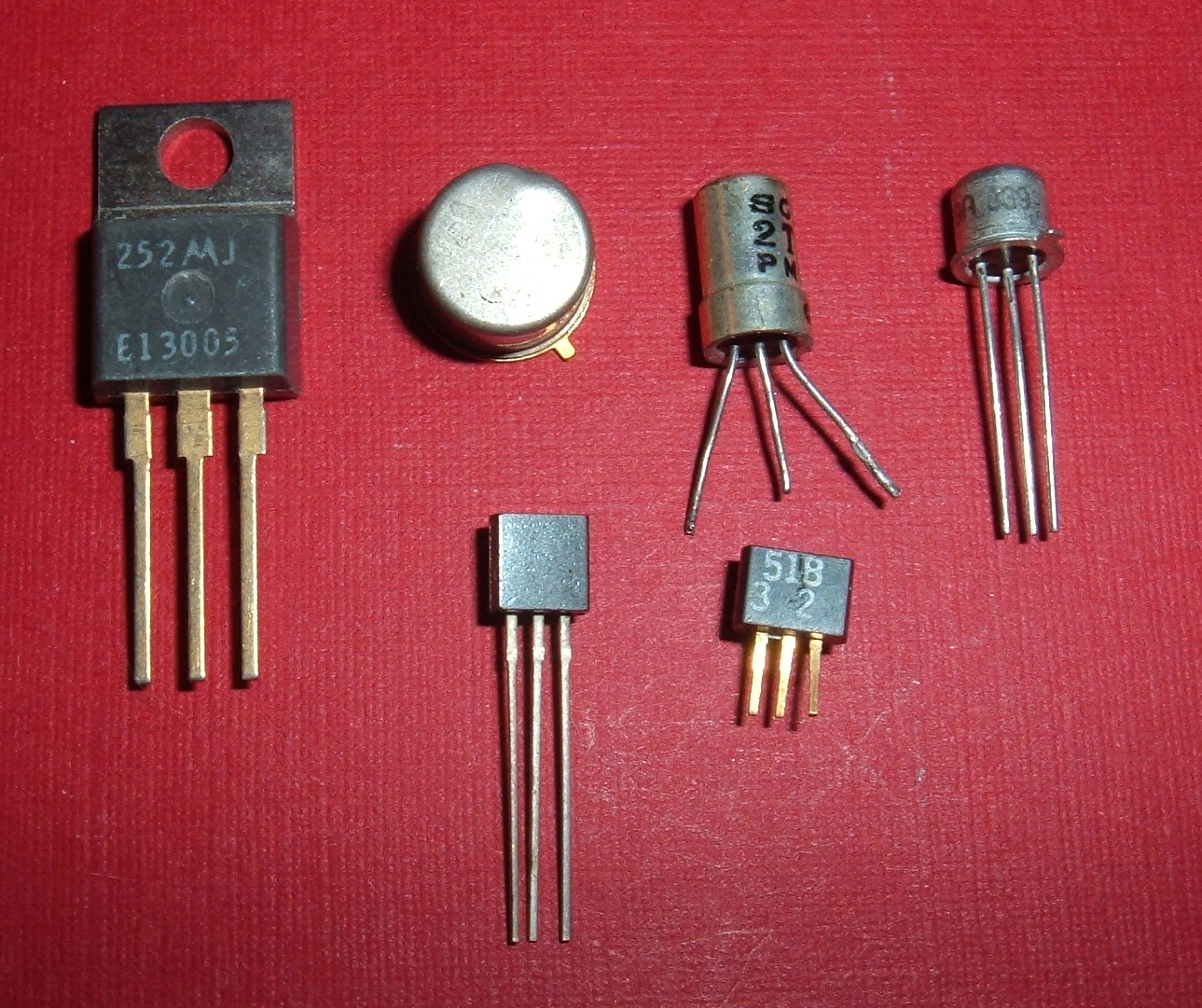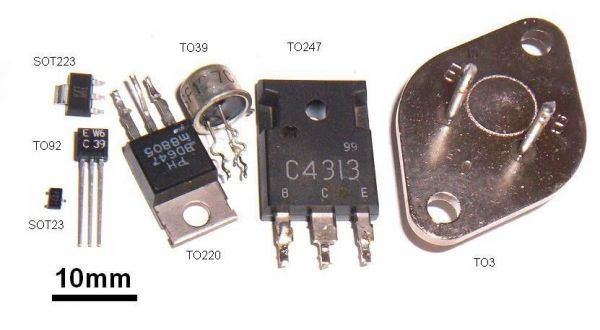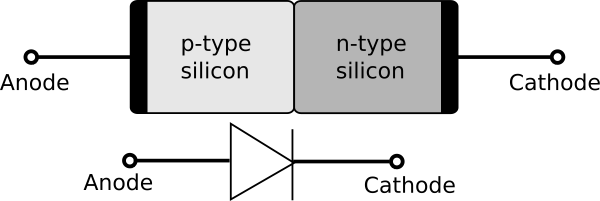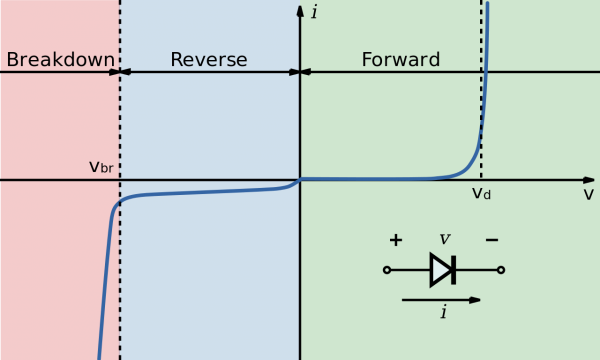# About transistors - Part 1 : the diode

I hate a lot of things, but I think the top one is website roughly teaching about transistors...

There are a lot - and I mean a LOT - of website talking about transistors. How they work, how they are used, how to bias them... Unfortunately there very few websites telling how to use and bias transistors correctly ! Let's explore a bit what is a transistor and how to work with them in this first series of article.

When it comes to audio, you'll mostly encouter three types of transistors : Bipolar Junction Transistors (BJT), Junction Field Effect Transistors (JFET) and Metal-Oxyde Semiconductor Field Effect Transistors (MOSFET). These types all behave differently, hence sound differently, and have different characteristics. However, the sound is not defined only by the type of transistor you use, but the structure you use. That's to say talking about BJT vs JFET is only useful when you specify the arrangement of the components in the circuit !

The thumbnail shows different through-hole transistors. Surface Mounted Devices (SMD) transistors are much smaller, but have lower temperature limit and are harder to solder. The following picture shows a size comparison.The first type of transistor I want to dissect is the BJT transistor. To fully understand how these transistors work, we shall first study a simpler component : the junction diode !

A diode is a dipole, a component with two electrodes : the anode and the cathode. A diode is made of a semiconductor material - often germanium or silicon - which is either enhanced or depleted in electron. Removing or adding electrons in a semiconductor is said to dope the semiconductor. Adding electrons is N-type doping, while removing electrons is P-type doping, hence the P-type is more positive than the N-type part of the diode, making the two poles : the anode is the P-type and the cathode is the N-type !Let's say you're using a battery to power the diode. If the cathode is connected to the positive pole of the battery, the electrons in it are attracted toward the battery. On the other side, the "loss of electrons", called holes, are attracted toward the other pole, because it is negative. Holes and electrons are attracted in opposite directions and "agglomerate" near each electrode of the diode. No current is flowing, the diode is blocking because the voltage accross the diode is negative. Note that the voltage is always measured from cathode to anode.

However, when you turn the battery around, this voltage turns positive. Electrons at the cathode are attracted to the anode, and holes in the anode are attracted to the cathode. If the voltage is high enough, the particles can flow in the circuit : a current is flowing, the diode is passing current ! This treshold voltage (Vd) depends on the material of the diode and temperature. Vd is around 0.3 V for germanium and 0.7 V for silicon.

Let's sum up how the diode works. When the voltage from cathode to anode is negative, the diode doesn't pass current. The diode is reverse biased. When this voltage is positive and higher than the treshold voltage, current is allowed to pass through the diode. It is forward biased. The more positive the voltage, the higher the current, but you should be careful not to burn the diode with too much power ! On the other side, if the voltage is too negative, the diode will break. It is the breakdown region of the diode.

To know how the current behaves with voltage, one can trace the I-V characteristics of the diode. The equation giving the amount of current when the diode is forward biased is the Shockley Equation. For our maths folks reading this article, it is just telling that the current in the diode is an exponential function of the voltage. Hence, when the current flowing through the diode is roughly constant, the voltage accross the diode is almost constant and equals Vd - first order approximation. It is important to understand this approximation, because it will be really useful when talking about the BJT transistor !## 1 comment

#1  - Gripsou said :

Transistors just saved my life. Thank you so much <3 !!!!